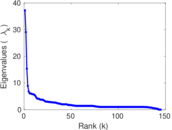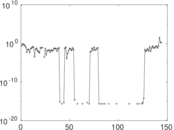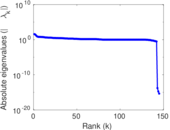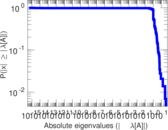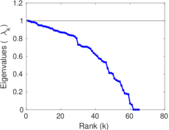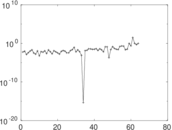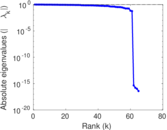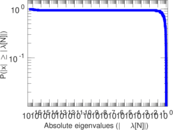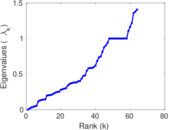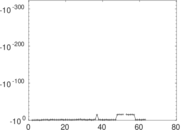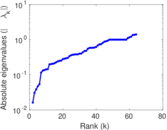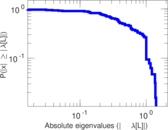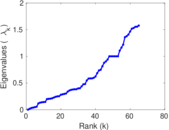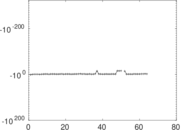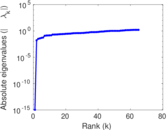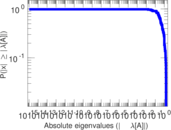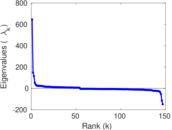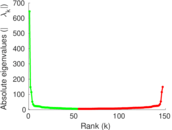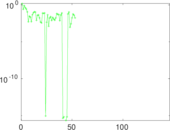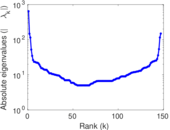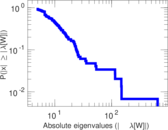# Wikiquote edits (wo)

This is the bipartite edit network of the Wolof Wikiquote. It contains users and pages from the Wolof Wikiquote, connected by edit events. Each edge represents an edit. The dataset includes the timestamp of each edit.

 Code `qwo` Internal name `edit-wowikiquote` Name Wikiquote edits (wo) Data source http://dumps.wikimedia.org/ AvailabilityDataset is available for download Consistency checkDataset passed all tests Category Authorship network Dataset timestamp 2017-10-20 Node meaning User, article Edge meaning Edit Network formatBipartite, undirected Edge typeUnweighted, multiple edges Temporal dataEdges are annotated with timestamps

## Statistics

 Size n = 654 Left size n1 = 147 Right size n2 = 507 Volume m = 841 Unique edge count m̿ = 637 Wedge count s = 20,079 Claw count z = 1,064,272 Cross count x = 46,820,407 Square count q = 383 4-Tour count T4 = 84,702 Maximum degree dmax = 198 Maximum left degree d1max = 198 Maximum right degree d2max = 39 Average degree d = 2.571 87 Average left degree d1 = 5.721 09 Average right degree d2 = 1.658 78 Fill p = 0.008 547 01 Average edge multiplicity m̃ = 1.320 25 Size of LCC N = 425 Diameter δ = 15 50-Percentile effective diameter δ0.5 = 4.617 27 90-Percentile effective diameter δ0.9 = 7.570 57 Median distance δM = 5 Mean distance δm = 5.006 30 Gini coefficient G = 0.601 247 Balanced inequality ratio P = 0.263 971 Left balanced inequality ratio P1 = 0.225 922 Right balanced inequality ratio P2 = 0.368 609 Relative edge distribution entropy Her = 0.858 784 Power law exponent γ = 4.845 23 Tail power law exponent γt = 2.591 00 Tail power law exponent with p γ3 = 2.591 00 p-value p = 0.049 000 0 Left tail power law exponent with p γ3,1 = 2.111 00 Left p-value p1 = 0.293 000 Right tail power law exponent with p γ3,2 = 3.201 00 Right p-value p2 = 0.154 000 Degree assortativity ρ = −0.263 622 Degree assortativity p-value pρ = 1.376 06 × 10−11 Spectral norm α = 37.229 0 Algebraic connectivity a = 0.016 192 8 Spectral separation |λ1[A] / λ2[A]| = 1.281 25 Controllability C = 359 Relative controllability Cr = 0.558 320

## Plots

### Fruchterman–Reingold graph drawing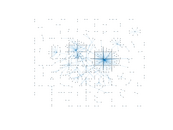### Degree distribution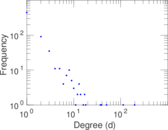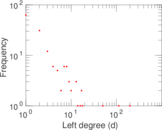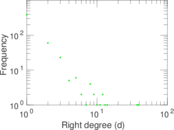### Cumulative degree distribution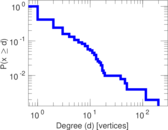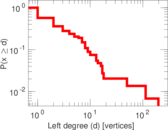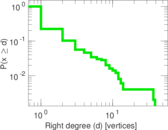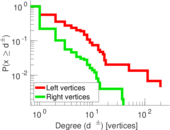### Lorenz curve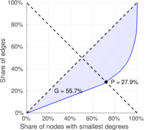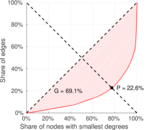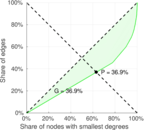### Spectral distribution of the adjacency matrix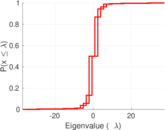### Spectral distribution of the normalized adjacency matrix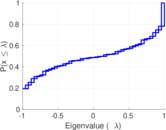### Spectral distribution of the Laplacian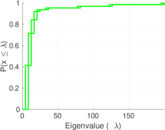### Spectral graph drawing based on the adjacency matrix### Spectral graph drawing based on the Laplacian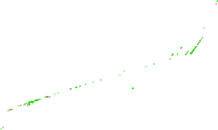### Spectral graph drawing based on the normalized adjacency matrix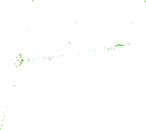### Degree assortativity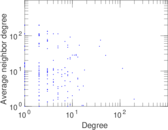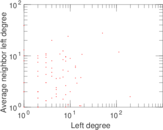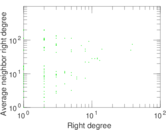### Zipf plot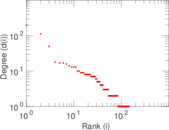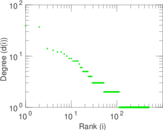### Hop distribution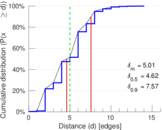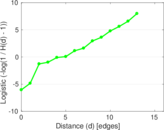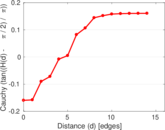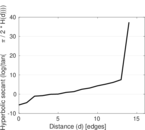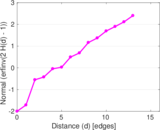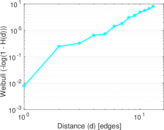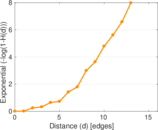### Double Laplacian graph drawing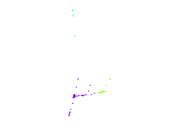### Delaunay graph drawing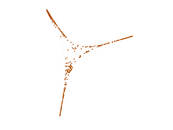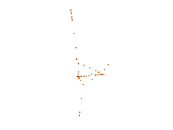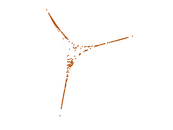### Edge weight/multiplicity distribution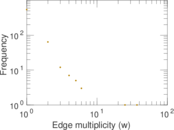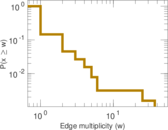### Temporal distribution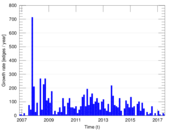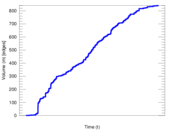### Temporal hop distribution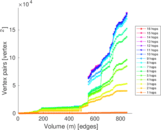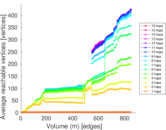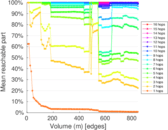### Diameter/density evolution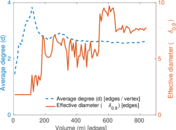### Matrix decompositions plots